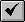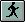HOW TO

# Convert a number field to a string field with commas using Avenue

## Summary

Use the script below to create a string field based on a number field so commas can be displayed.

## Procedure

1. Open a new script window.

A. Activate the Project window.
B. Click the Scripts icon.
C. Click New.

2. Copy the code into the new script window.

`Code:'This script assumes that a table is the active document'It will prompt you for the numeric field that you want to 'convert to a string field with commas.'It will also ask you for a name for the new string field'The script will put your table in edit mode, add the new field,'populate the field and then save the changes and stop editing.aTable = av.GetActiveDoc  'Gets active tableaVtab = aTable.GetVtab flds = aVtab.GetFields f_List = List.Make for each f in flds   if (f.IsTypeNumber) Then     nf = f_List.Add(f)   end end select = MsgBox.ListAsString(f_List,"Select a Number Field","Selection") newFieldName = MsgBox.Input("Please input a name for the new string field:", "New Field","Comma")newfld = Field.Make(newFieldName,#FIELD_CHAR,20,0) aVtab.SetEditable(true) aVtab.AddFields({newfld}) For each rec in aVtab   aVal = aVtab.ReturnValueNumber(select,rec)   'MsgBox.Info(aVal.AsString,"aVal")     com = ","     c = aVal.AsString.Count     If (c = 4) Then     num = (aVal.AsString.Left(1))+(com+aVal.AsString.Right(3))     ElseIf (c = 5) Then     num = (aVal.AsString.Left(2))+(com+aVal.AsString.Right(3))     ElseIf (c = 6) Then     num = (aVal.AsString.Left(3))+(com+aVal.AsString.Right(3))     ElseIf (c = 7) Then     num =     (aVal.AsString.Left(1))+(com+aVal.AsString.Middle(1,3))+(com+aVal.AsString.Right(3))     ElseIf (c = 8) Then     num =     (aVal.AsString.Left(2))+(com+aVal.AsString.Middle(2,3))+(com+aVal.AsString.Right(3))     ElseIf (c = 9) Then     num =     (aVal.AsString.Left(3))+(com+aVal.AsString.Middle(3,3))+(com+aVal.AsString.Right(3))     else     num = aVal.AsString   end 'end If Then    aVtab.SetValue(newfld,rec,num) end 'For aVtab.SetEditable(false)`

3. Compile the script by clicking the compile button.4. Run the script by clicking the run button.Article ID:000005042

Software:
• Legacy Products

Get help from ArcGIS experts

Contact technical support